NOTIFICATION: These examples are provided for educational purposes. The use of this code and/or information is under your own responsibility and risk. The information and/or code is given ‘as is’. I do not take responsibilities of how they are used.

Symmetric Encryption

In the symmetric encryption, the same key (normally a single-key) is used to perform the encryption and decryption of the ciphertext.

Symmetric Cipher Model: This model is performed by performing transformations and substitutions on the plaintext. A secret key, independent from the plaintext and the algorithm, is used to cipher the plaintext. After, the ciphertext plus the secret key is used with the decryption algorithm to obtain the original plaintext.

Symmetric Encryption is the opposite to the concept of public key distribution which will be explained in future postings.

Requirements:

1. The cipher model must be mathematical expression:
(E: Encryption, D: Decryption, X: plaintext, Y: ciphertext, K: secret key)

```Y = E(K, X)
X = D(K, Y)
```
2. Assumption that the encryption algorithm is known to the attacker.
3. A strong encryption algorithm which in case the attacker would obtain or know some examples of the ciphertext and the plaintext produced from the ciphertext, the attacker would still be not able to obtain the key. This means that if the attacker would obtain the ciphertext, the attacker would not be able to obtain the secret key or the plain text.
4. Secret key should be known only by the sender and the receiver of the ciphertext.
5. The distribution of the secret key must be done in a secure fashion. For example, the use of a third party that would generate and provide in a secure way the key to the sender and the receiver.

Substitution Ciphers

In classical substitution ciphers, all the letters in the plaintext will be replaced by another letter, number, and/or symbol.

Caesar Cipher

History explains that Julius Caesar <http://www.roman-empire.net/republic/caesar-index.html> came up with a substitution cipher that he used in his campaigns for military affairs.

The cipher works in the following way:

1. We use the alphabet of 26 letters:
```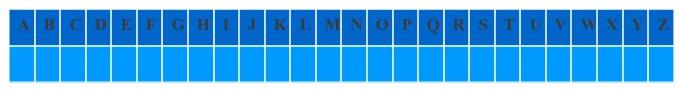```
2. Under this alphabet, we will rewrite the alphabet by picking a letter as a starting point.
Lets say our key indicate the starting point such as K = 4 so we begin with the letter ‘E’ then: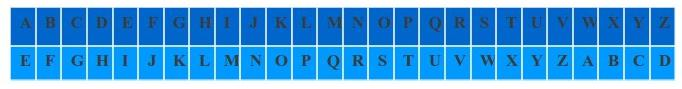3. This means that if we wish to send a plaintext (P) that says HELLO, the ciphertext (C) would be LIPPS, and the key (K) would be 4
4. The mathematical way to represent this cipher will be the follows:
1. Give each letter of the alphabet a number:
A = 1, B = 2, C = 3, D = 4, E = 5,F = 6, G = 7, H = 8, J = 9, K = 10, L = 11, M = 12, N = 13, O = 14,P = 15, Q = 16, R = 17, S = 18, T = 19, U = 20, V = 21, W = 22, X = 23, Y = 24, Z = 25.
2. Encryption Algorithm:
E: Encryption, Ct: Ciphertext, Pt: Plaintext, K: secret key

```Ct = E(Pt)
= (Pt + K) mod 26```
3. Decryption Algorithm:
D: Decryptor, Ct: Ciphertext, Pt: Plaintext, K: secret key

```Pt = D(Ct)
= (26 + (Ct - K)) mod 26```
5. The weakness of this cipher is that it can be broken by brute force. We just need to test the 25 combinations of different keys  until we find the key that reveals the message.

Monoalphabetic Cipher

The mono-alphabetic cipher instead of shifting the alphabet a number of letters, its substitute each letter arbitrarily by mapping the plaintext letter map to a random arranged ciphertext. The only requirement for the ciphertext is that the letters must not be repeated.

Since we are using 26 letters of the alphabet the arrangement of the cipher can permute a total of 26! permutations.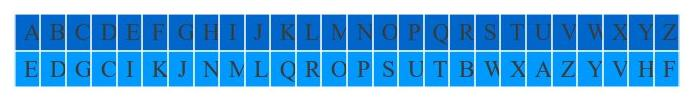If we wish to encode the word “HELLO”, we would obtain “NERRS”

Lets assume we wish to cipher a plaintext:

Plaintext = “THIS IS A SECRET MESSAGE ENCODED IN MONOALPHABETIC”

Ciphertext = “XNMW MW E WIGBIX OIWWEJI IPGSCIC MP OSPSERUEDIXMG”

The following website let you play a little with monoalphabetic cipher by randomizing for you the ciphertext:

The only problem is that this cipher can be exploited by doing regularities analysis over the frequency of the letters. Base on the language rules some letters are used more than others. For example, in English, the letter ‘E’ is the most common used in words, followed by A, I, O, N, R, S, T. Others letters such as K, J, Q, X, Z are less used than the rest.

The largest is the message, the most chances that the attacker can decrypt the message.
Just in this message “XNMW MW E WIGBIX OIWWEJI IPGSCIC MP OSPSERUEDIXMG” we have:

• W = 6 letters
• E = 4 letters
• M = 4 letters
• S = 3 letters
• P = 2 letters
• ….

And continue counting.

As you may notice the letter ‘W’ of the encrypted message have the most counts, so we could  assume that this is the letter E of the plaintext.

If you are interested to know the frequency of letters in English you can go to the following website:
<http://www.cryptograms.org/letter-frequencies.php>

<http://unsecure.co.uk/attackingmonoalphabeticciphers.asp>

Playfair Cipher

Playfair is one way to improve the security of mono-alphabetic cipher by encrypting multiple letters.

Playfair Encryption

1. Create a playfair key matrix:
1. Create a matrix of letters based on a keyword. For this example, the matrix should be 5 by 5
2. Fill in the letters of the keyword from left to right and from top to bottom. Make sure that there are not duplicate letters
3. Fill the rest of the matrix with the other letters that are not in the keyboard, making sure to not duplicate letters.
4. As a rule, the letter I and J count as one letter.
• I am not sure the reason for this rule, except the following:
• First, it make it harder to decrypt the message since one letter is missing.
• Second, in some languages, the J and I would have the same pronunciation.
For example, my last name Carlstein was originally written as Karlštejn.
• In case you know the real reason, please let me know and give me a reference to verify (thanks).
5. Example of playfair key matrix:
1. Let use the keyword: “EDUCATOR”
2. The table should looks like this: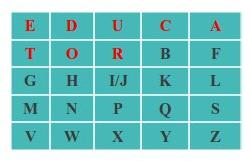3. Notice that I and J are counted as one letter
2. The next step is to encrypt the plaintext taking two letters at the time.
1. In case a two letters are the same (repeated), we must insert a filler letter (use the letter X as the filler). For example:
HELLO → HE LX LO
2. In case two letters are in the same row, replace each letter with the letter to the right. In case the letter is at the last column, pick the letter of the first row (the table is considerate to be circular). For example, lets say we have the letters D and A:1. D → U and A → E
2. Therefore DA became UE
3. In case two letters are in the same column, replace each letter with the letter below. In case the letter is at the last row, pick the letter of the first row (the table is considerate to be circular). For example, lets say we have the letters T and V:1. T → G and V → E
2. Therefore TV became GE
4. In case two letter are in different row and column, the first letter will be replaced with another letter of the same row on the column of the second letter. The second letter will be replaced with another letter of the same row on the column of the first letter. For example lets say we have the letters O and Q: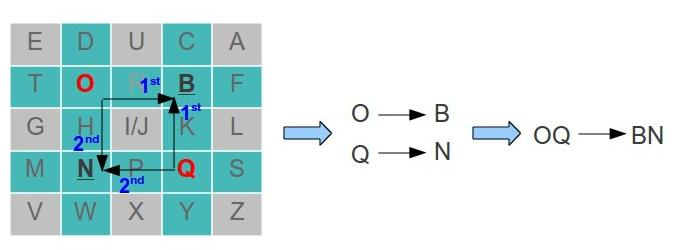1. To replace the letter O:
1. This means that O → B
2. To replace the letter Q:
1. This means that Q → N
3. Therefore OQ became BN

Playfair Decryption:

1. Decrypt two letters at a time:
1. In case two letters are in the same row, replace each letter with the letter to the left. In case the letter is at the last column, pick the letter of the first row (the table is considerate to be circular). For example, lets say we have the letters U and E: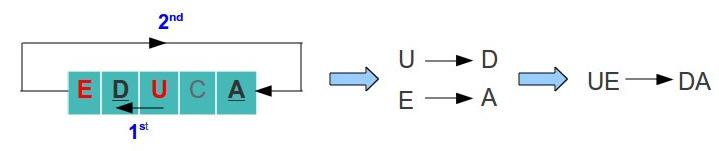1. U → D and E → A
2. Therefore UE became DA
2. In case two letters are in the same column, replace each letter with the letter above. In case the letter is at the last row, pick the letter of the first row (the table is considerate to be circular). For example, lets say we have the letters G and E: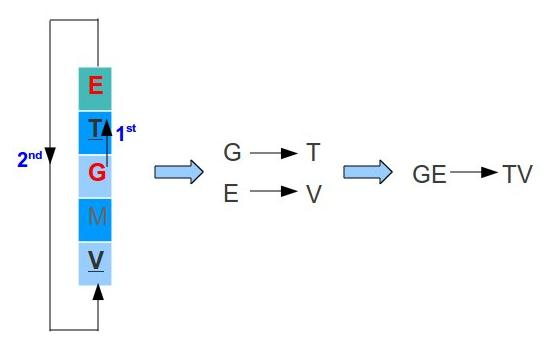1. G → T and E → V
2. Therefore GE became TV
3. In case two letter are in different row and column, the first letter will be replaced with another letter of the same row on the column of the second letter. The second letter will be replaced with another letter of the same row on the column of the first letter. For example lets say we have the letters B and N: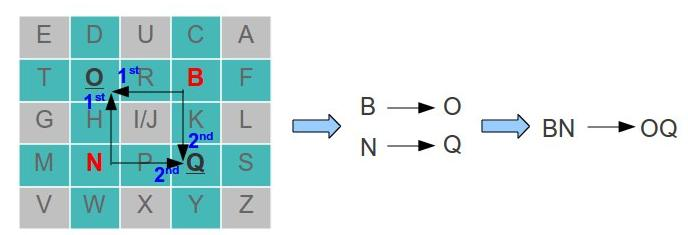1. To replace the letter B:
1. This means that O → B
2. To replace the letter Q:
1. This means that Q → N
3. Therefore OQ became BN
2. After you will finish with the final message. You must remove any extra X that do not make sense in the message:
HE LX LO → HELLO

1. Thanks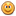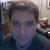Alejandro G. Carlstein Ramos Mejia